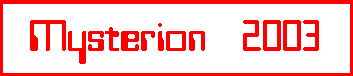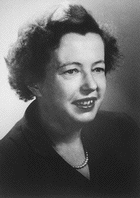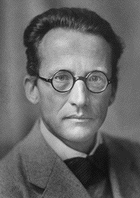# Nuclear Term Schemata and Magic Nucleon Numbers

 * Structures in Micro Objects *

Nuclear Term SchemaThe nuclear term schema for an arbitrary nuclide
is obtained by solving the Schrödinger equation
for a modified 3-dimensional harmonic oscillator potential.

Taking into account the spin orbit interaction of angular momentum,
Maria Goeppert-Mayer succeeded in analyzing the structure
of the nuclear shells, interpreting the magic occupation numbers,
and showing that protons and neutrons have slightly different higher terms.
For any nucleon state, we denote by
n ... the principal quantum number (oscillator shells with n = 1,2,...),
l ... the orbital angular momentum (orbitals s,p,... with l = 0,...,n),
s ... the spin quantum number (s = -l/2 or +1/2),
j ... the total angular momentum.

Boldface state numbers denote closed neutron shells
and are called nuclear magic numbers.
The schema for protons is similar up to occupation number 82
but differs in higher regions.

# of statess (l = 0)p (l = 1)d (l = 2)f (l = 3)g (l = 4)h (l = 5)i (l = 6)j (l = 7)k (l = 8)
184___3d(3/2)4
180___4s(1/2)2
178___2g(7/2)8
170___1j(15/2)16
154___1i(11/2)12
142___3d(5/2)6
136___2g(9/2)10
126___2p(1/2)2
124___2p(3/2)4
120___1i(13/2)14
106___2f(5/2)6
100___2f(7/2)8
92___1h(9/2)10
82___3s(1/2)2
80___2d(3/2)4
76___1h(11/2)12
64___2d(5/2)6
58___1g(7/2)8
50___1g(9/2)10
40___2p(1/2)2
38___1f(5/2)6
32___2p(3/2)4
28___1f(7/2)8
20___1d(3/2)4
16___2s(1/2)2
14___1d(5/2)6
8___1p(1/2)2
6___1p(3/2)4
2___1s(1/2)2
Magic Nucleon Numbers
The table below lists the few stable nuclides with doubly magic nucleon numbers.

 2 He 4 8 O 16 20 Ca 40 20 Ca 48 82 Pb 208 114 Uuq 298 164 Uhq 482

References:
Rod Nave, Georgia State University
Jim Branson, University of California, San Diego

 <| Navigation Center <| <| Back to Algebra<| <| Back to Curriculum<|